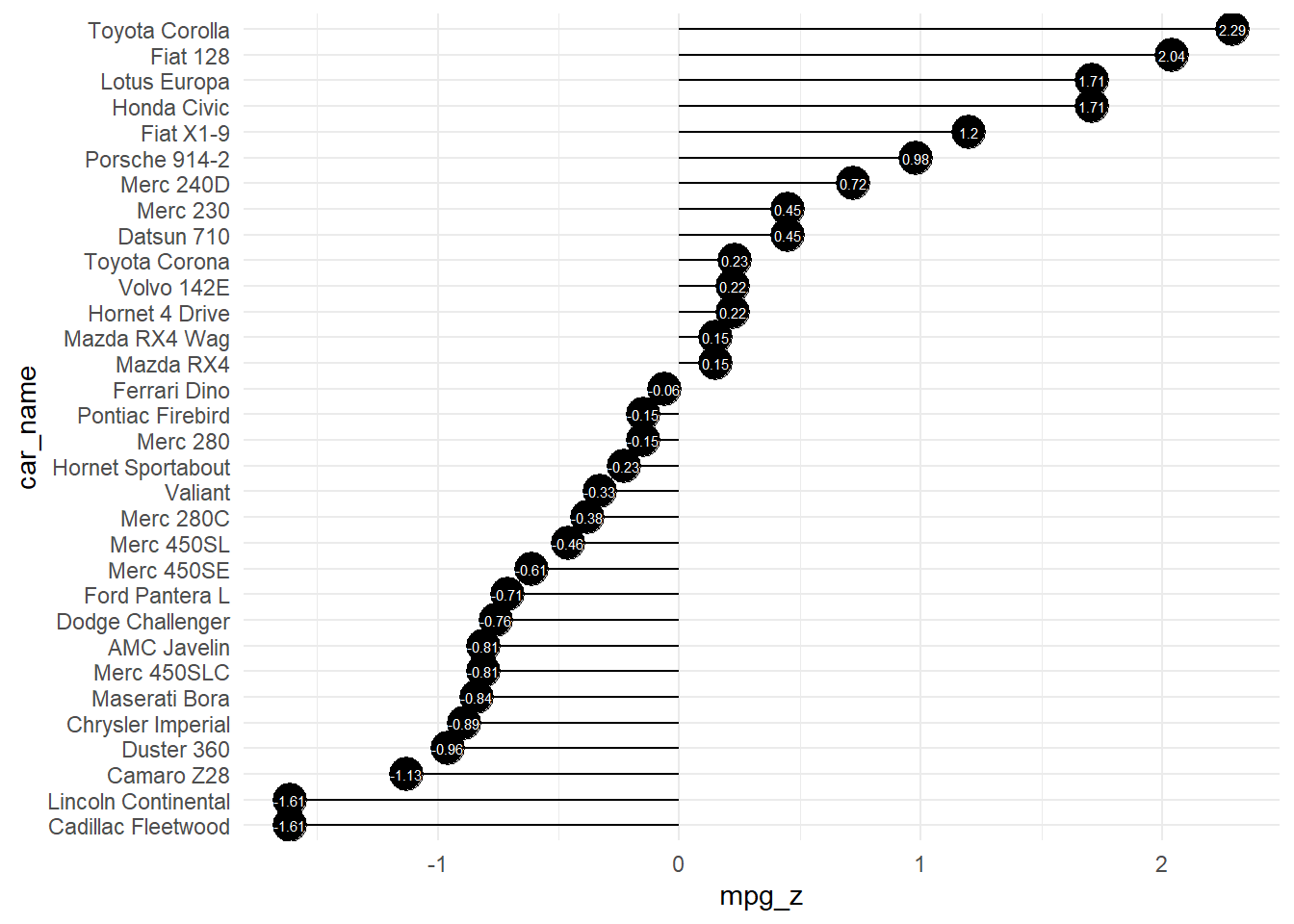# Diverging Lollipop Chart: A Visual Tool for Comparing Data with {healthyR}

code
rtip
healthyr
plots
Author

Steven P. Sanderson II, MPH

Published

February 2, 2023

# Introduction

A diverging lollipop chart is a useful tool for comparing data that falls into two categories, usually indicated by different colors. This type of chart is particularly well-suited for comparing the differences between two data sets and for identifying which data points are contributing most to the differences.

The R package `{healthyR}` offers a function called `diverging_lollipop_plt()` that can be used to create a diverging lollipop chart. This function has several parameters that can be used to customize the chart to meet your specific needs.

In conclusion, the diverging lollipop chart is a useful tool for comparing data sets and can provide insights into the differences between two sets of data. The `diverging_lollipop_plt()` function from the `{healthyR}` package is a great option for creating this type of chart, as it offers a range of customization options to meet your specific needs. Whether you’re working with data related to business, finance, or any other field, a diverging lollipop chart can be a valuable tool in your visual analysis toolkit.

# Function

Let’s take a look at the full function call.

``````diverging_lollipop_plt(
.data,
.x_axis,
.y_axis,
.plot_title = NULL,
.plot_subtitle = NULL,
.plot_caption = NULL,
.interactive = FALSE
)``````

Now lets see the arguments that get provided to the parameters.

• `.data` - The data to pass to the function, must be a tibble/data.frame.
• `.x_axis` - The data that is passed to the x-axis. This will also be the x and xend parameters of the geom_segment
• `.y_axis` - The data that is passed to the y-axis. This will also equal the parameters of `yend` and `label`
• `.plot_title` - Default is NULL
• `.plot_subtitle` - Default is NULL
• `.plot_caption` - Default is NULL
• `.interactive` - Default is FALSE. TRUE returns a plotly plot

# Example

Let’s see an example.

``````library(healthyR)

suppressPackageStartupMessages(library(ggplot2))

data("mtcars")
mtcars\$car_name <- rownames(mtcars)
mtcars\$mpg_z <- round((mtcars\$mpg - mean(mtcars\$mpg))/sd(mtcars\$mpg), 2)
mtcars\$mpg_type <- ifelse(mtcars\$mpg_z < 0, "below", "above")
mtcars <- mtcars[order(mtcars\$mpg_z), ]  # sort
mtcars\$car_name <- factor(mtcars\$car_name, levels = mtcars\$car_name)

diverging_lollipop_plt(
.data = mtcars,
.x_axis = car_name,
.y_axis = mpg_z
)``````Now let’s also see the interactive chart.

``````diverging_lollipop_plt(
.data = mtcars,
.x_axis = car_name,
.y_axis = mpg_z,
.interactive = TRUE
)``````

Voila!